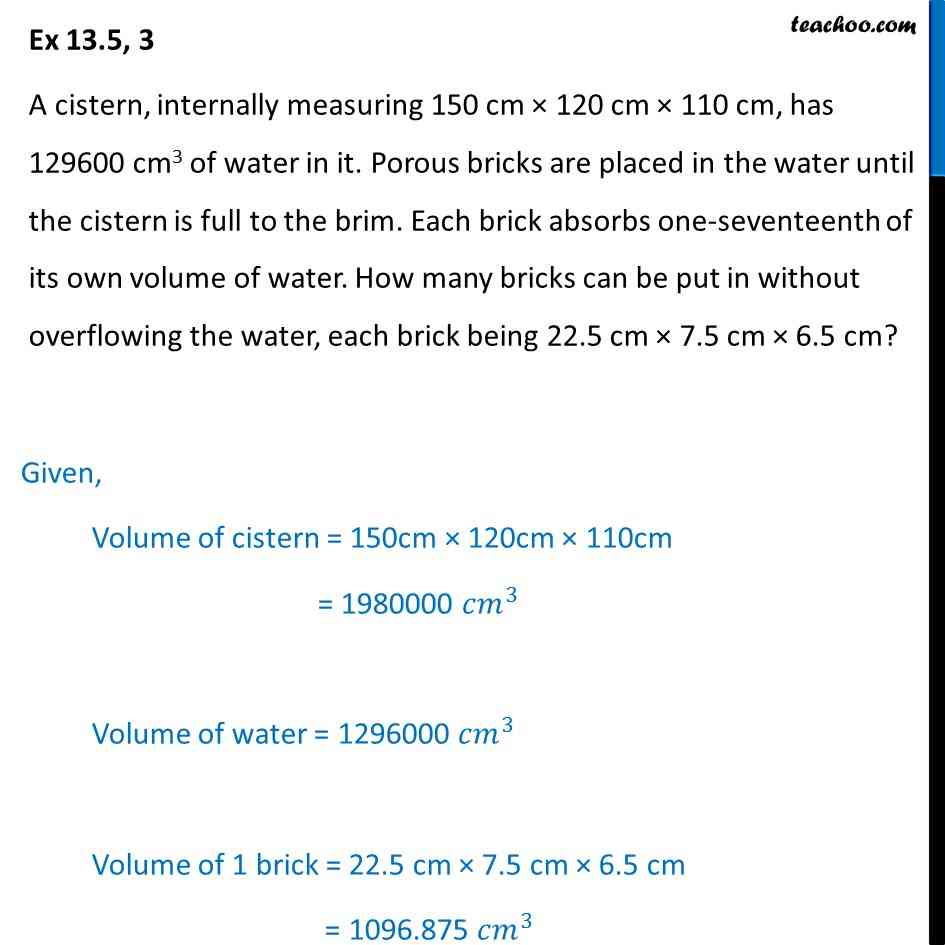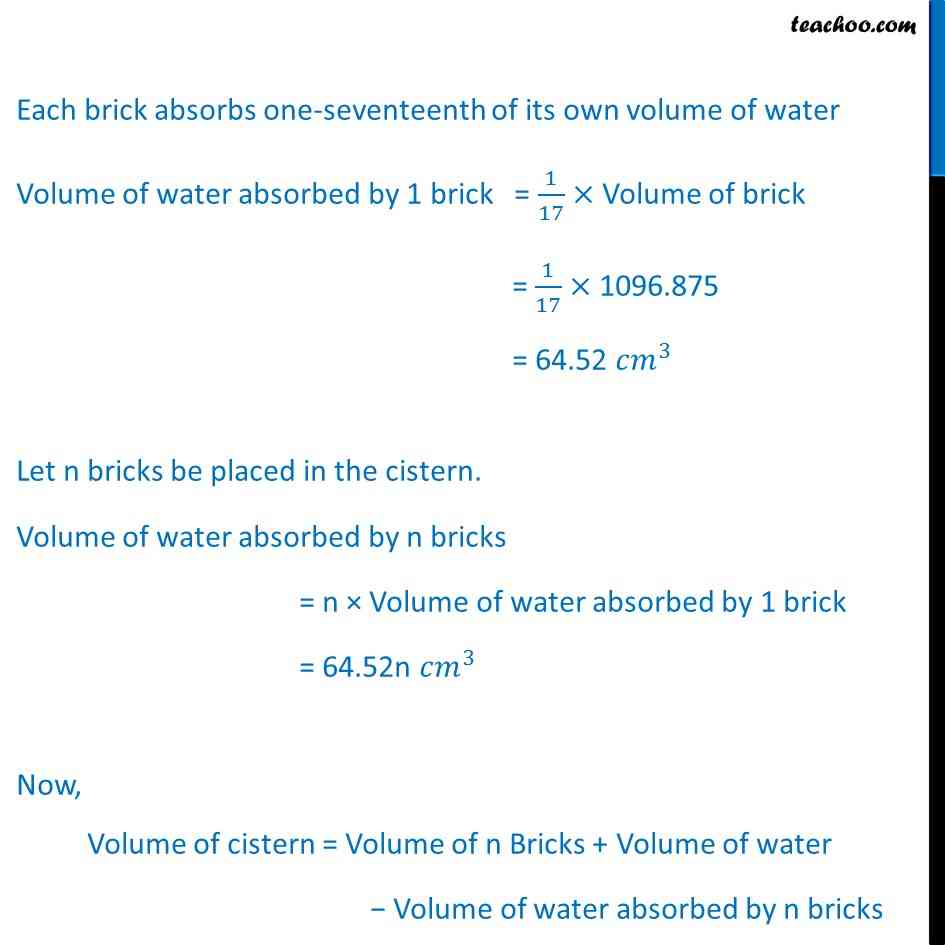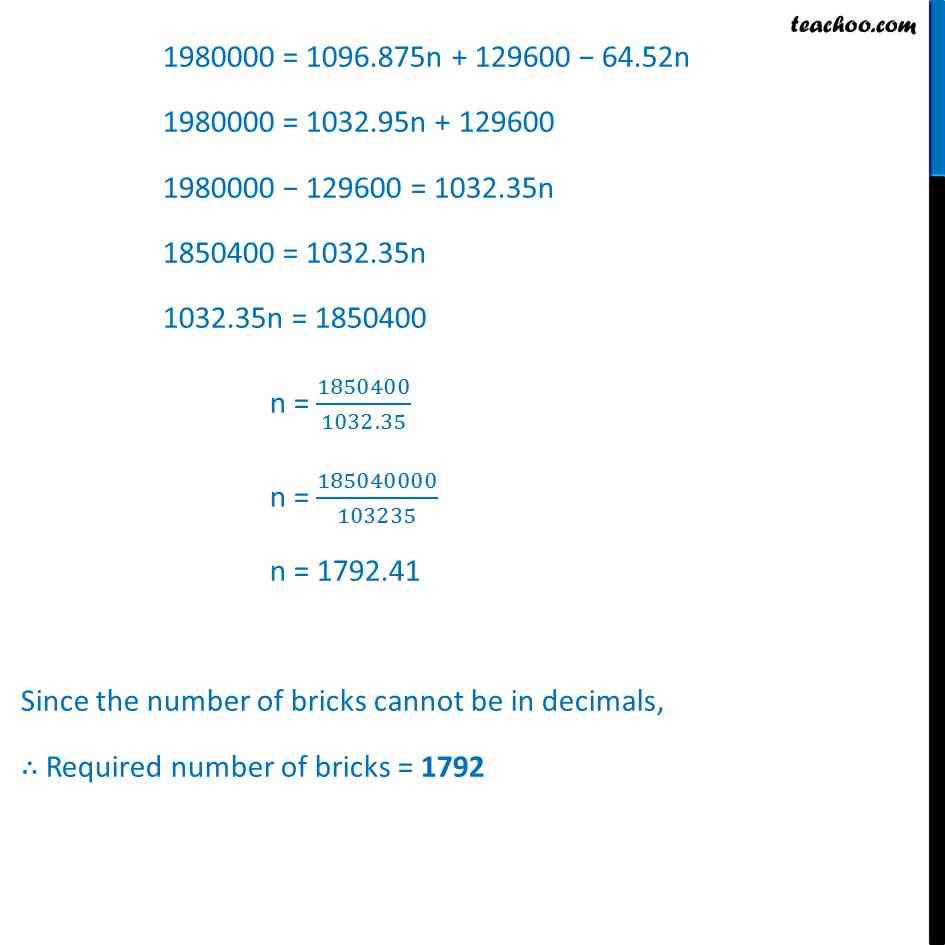Ex 13.5 (Optional)

Chapter 13 Class 10 Surface Areas and Volumes
Serial order wiseGet live Maths 1-on-1 Classs - Class 6 to 12

### Transcript

Ex 13.5, 3 A cistern, internally measuring 150 cm × 120 cm × 110 cm, has 129600 cm3 of water in it. Porous bricks are placed in the water until the cistern is full to the brim. Each brick absorbs one-seventeenth of its own volume of water. How many bricks can be put in without overflowing the water, each brick being 22.5 cm × 7.5 cm × 6.5 cm? Given, Volume of cistern = 150cm × 120cm × 110cm = 1980000 〖𝑐𝑚〗^3 Volume of water = 1296000 〖𝑐𝑚〗^3 Volume of 1 brick = 22.5 cm × 7.5 cm × 6.5 cm = 1096.875 〖𝑐𝑚〗^3 Each brick absorbs one-seventeenth of its own volume of water Volume of water absorbed by 1 brick = 1/17× Volume of brick = 1/17× 1096.875 = 64.52 〖𝑐𝑚〗^3 Let n bricks be placed in the cistern. Volume of water absorbed by n bricks = n × Volume of water absorbed by 1 brick = 64.52n 〖𝑐𝑚〗^3 Now, Volume of cistern = Volume of n Bricks + Volume of water − Volume of water absorbed by n bricks 1980000 = 1096.875n + 129600 − 64.52n 1980000 = 1032.95n + 129600 1980000 − 129600 = 1032.35n 1850400 = 1032.35n 1032.35n = 1850400 n = 1850400/1032.35 n = 185040000/103235 n = 1792.41 Since the number of bricks cannot be in decimals, ∴ Required number of bricks = 1792